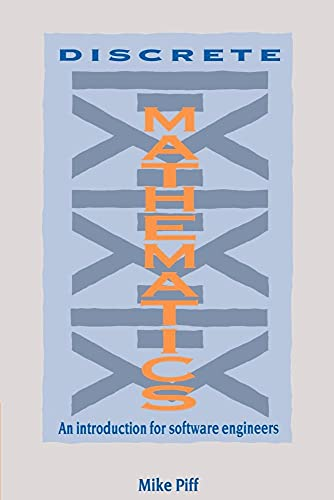# Discrete Mathematics by Mike Piff (University of Sheffield)

In Stock
£3.99
This book is designed to form the basis of a one-year course in discrete mathematics for first-year computer scientists and software engineers. It covers much of undergraduate algebra with a bias towards computing applications. It includes: mathematical logic; set theory, finite and infinite relations and mappings; graphs; graphical algorithms and axiom systems.
Only 3 left

## Discrete Mathematics Summary

### Discrete Mathematics: An Introduction for Software Engineers by Mike Piff (University of Sheffield)

Computing developed as a branch of mathematics, only to drift away from this home as computer science diverged towards more general topics such as the theory of how a computer works. Recently the emphasis has become more mathematical and the new disciplines of software engineering and information technology have emerged. This book is designed to form the basis of a one year course in discrete mathematics for first year computer scientists or software engineers. The material presented covers much of undergraduate algebra with a particular bias towards the computing applications. Topics covered include mathematical logic, set theory, finite and infinite relations and mappings, graphs, graphical algorithms and axiom systems. It concludes with implementations of many of the algorithms in Modula-2 to illustrate how the mathematics may be turned into concrete calculations. Numerous examples and exercises are included with selected solutions to the problems appearing in the appendix. Discrete mathematics is the basic language which every student of computing should take pride in mastering and this book should prove an essential tool in this aim.

### Why buy from World of BooksOur excellent value books literally don't cost the earthFree delivery in the UKEvery used book bought is one saved from landfill

## Discrete Mathematics Reviews

"A useful refresher for those who wish to refamiliarize themselves with the material." Choice
"...a very good freshman-level discrete math textbook." Mukkai S. Krishnamoorthy, Mathematical Reviews

1. Introduction; 2. Logic; 3. Set theory; 4. Relations; 5. Mappings; 6. Graphs; 7. Graph algorithms; 8. Abstract algebra; Appendices.

GOR001395306
Discrete Mathematics: An Introduction for Software Engineers by Mike Piff (University of Sheffield)
Mike Piff (University of Sheffield)
Used - Very Good
Paperback
Cambridge University Press
1991-06-27
332
0521386225
9780521386227
N/A
Book picture is for illustrative purposes only, actual binding, cover or edition may vary.
This is a used book - there is no escaping the fact it has been read by someone else and it will show signs of wear and previous use. Overall we expect it to be in very good condition, but if you are not entirely satisfied please get in touch with us.